# Vue双向绑定原理，教你一步一步实现双向绑定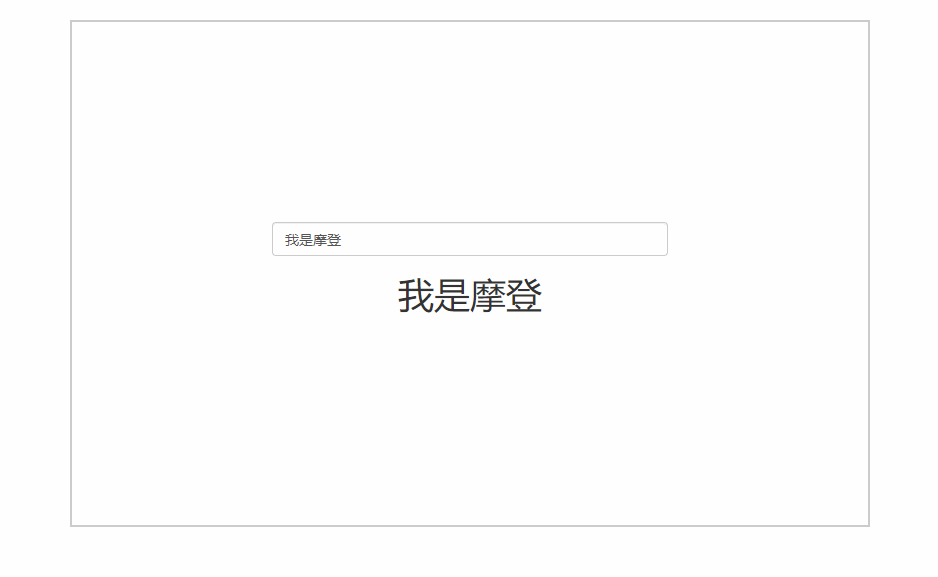//代码：
<div id="app">
<input v-model="name" type="text">
<h1>{{name}}</h1>
</div>
<script src="./js/observer.js"></script>
<script src="./js/watcher.js"></script>
<script src="./js/compile.js"></script>
<script src="./js/index.js"></script>
<script>
const vm = new Mvue({
el: "#app",
data: {
name: "我是摩登"
}
});
</script>
 

#### 数据绑定

• 发布订阅模式
• Angular 的脏查机制
• 数据劫持

#### Object.defineProperty

var people = {
name: "Modeng",
age: 18
}
people.age; //18
people.age = 20;

var modeng = {}
var age;
Object.defineProperty(modeng, 'age', {
get: function () {
console.log("获取年龄");
return age;
},
set: function (newVal) {
console.log("设置年龄");
age = newVal;
}
});
modeng.age = 18;
console.log(modeng.age);

#### 分析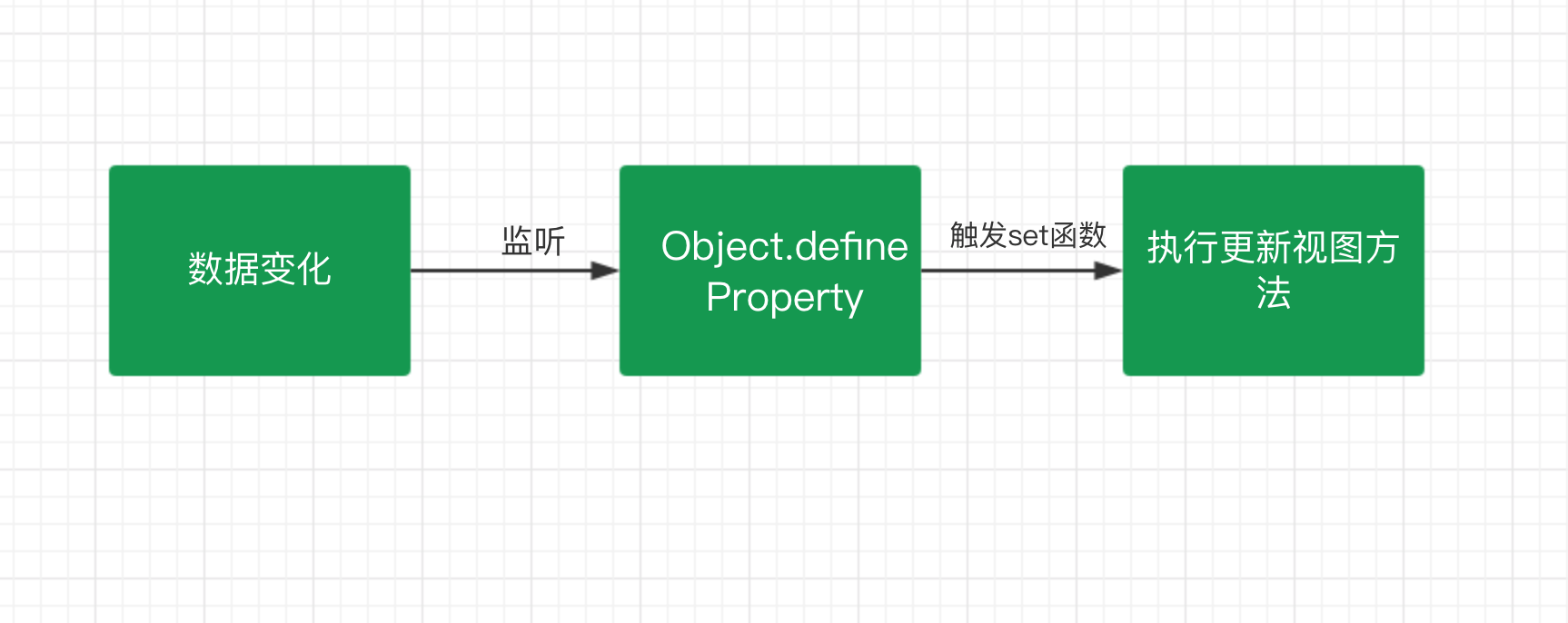#### 实现

• Observer 监听器：用来监听属性的变化通知订阅者
• Watcher 订阅者：收到属性的变化，然后更新视图
• Compile 解析器：解析指令，初始化模版，绑定订阅者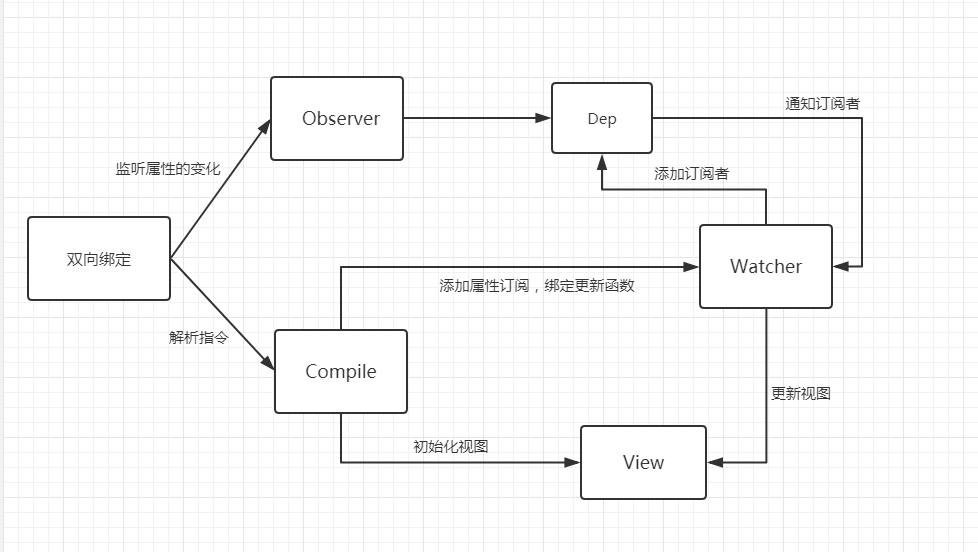#### 监听器 Observer

function defineReactive(data, key, value) {
//递归调用，监听所有属性
observer(value);
var dep = new Dep();
Object.defineProperty(data, key, {
get: function () {
if (Dep.target) {
}
return value;
},
set: function (newVal) {
if (value !== newVal) {
value = newVal;
dep.notify(); //通知订阅器
}
}
});
}

function observer(data) {
if (!data || typeof data !== "object") {
return;
}
Object.keys(data).forEach(key => {
defineReactive(data, key, data[key]);
});
}

function Dep() {
this.subs = [];
}
this.subs.push(sub);
}
Dep.prototype.notify = function () {
console.log('属性变化通知 Watcher 执行更新视图函数');
this.subs.forEach(sub => {
sub.update();
})
}
Dep.target = null;

 

var modeng = {
age: 18
}
observer(modeng);
modeng.age = 20;
 

#### 订阅者 Watcher

Watcher 主要是接受属性变化的通知，然后去执行更新函数去更新视图，所以我们做的主要是有两步：

1. 把 Watcher 添加到 Dep 容器中，这里我们用到了 监听器的 get 函数
2. 接收到通知，执行更新函数。
function Watcher(vm, prop, callback) {
this.vm = vm;
this.prop = prop;
this.callback = callback;
this.value = this.get();
}
Watcher.prototype = {
update: function () {
const value = this.vm.$data[this.prop]; const oldVal = this.value; if (value !== oldVal) { this.value = value; this.callback(value); } }, get: function () { Dep.target = this; //储存订阅器 const value = this.vm.$data[this.prop]; //因为属性被监听，这一步会执行监听器里的 get方法
Dep.target = null;
return value;
}
}
 

function Mvue(options, prop) {
this.$options = options; this.$data = options.data;
this.$prop = prop; this.$el = document.querySelector(options.el);
this.init();
}
Mvue.prototype.init = function () {
observer(this.$data); this.$el.textContent = this.$data[this.$prop];
new Watcher(this, this.$prop, value => { this.$el.textContent = value;
});
}
 

<div id="app">{{name}}</div>
const vm = new Mvue({
el: "#app",
data: {
name: "我是摩登"
}
}, "name");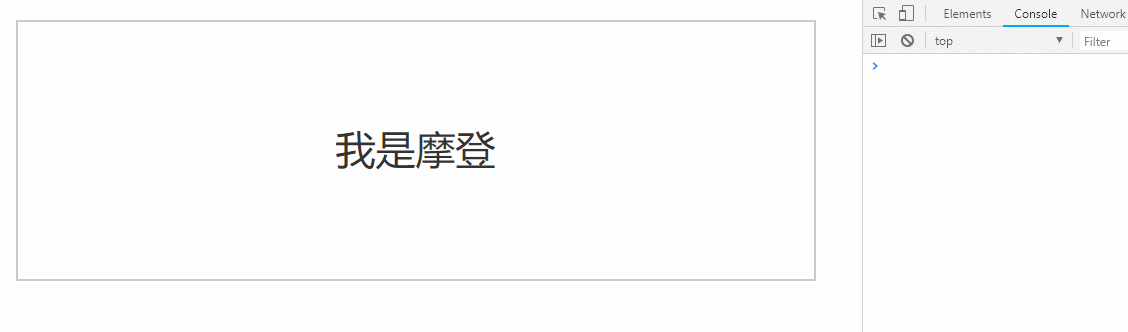#### Compile 解析器

Compile 的主要作用一个是用来解析指令初始化模板，一个是用来添加添加订阅者，绑定更新函数。

function Compile(vm) {
this.vm = vm;
this.el = vm.$el; this.fragment = null; this.init(); } Compile.prototype = { init: function () { this.fragment = this.nodeFragment(this.el); }, nodeFragment: function (el) { const fragment = document.createDocumentFragment(); let child = el.firstChild; //将子节点，全部移动文档片段里 while (child) { fragment.appendChild(child); child = el.firstChild; } return fragment; } }   然后我们就需要对整个节点和指令进行处理编译，根据不同的节点去调用不同的渲染函数，绑定更新函数，编译完成之后，再把 DOM 片段添加到页面中。 Compile.prototype = { compileNode: function (fragment) { let childNodes = fragment.childNodes; [...childNodes].forEach(node => { let reg = /\{\{(.*)\}\}/; let text = node.textContent; if (this.isElementNode(node)) { this.compile(node); //渲染指令模板 } else if (this.isTextNode(node) && reg.test(text)) { let prop = RegExp.$1;
this.compileText(node, prop); //渲染{{}} 模板
}

//递归编译子节点
if (node.childNodes && node.childNodes.length) {
this.compileNode(node);
}
});
},
compile: function (node) {
let nodeAttrs = node.attributes;
[...nodeAttrs].forEach(attr => {
let name = attr.name;
if (this.isDirective(name)) {
let value = attr.value;
if (name === "v-model") {
this.compileModel(node, value);
}
node.removeAttribute(name);
}
});
},
//省略。。。
}
 

#### 创建 Mvue

function Mvue(options) {
this.$options = options; this.$data = options.data;
this.$el = document.querySelector(options.el); this.init(); } Mvue.prototype.init = function () { observer(this.$data);
new Compile(this);
}
 

<div id="app">
<h1>{{name}}</h1>
</div>
<script src="./js/observer.js"></script>
<script src="./js/watcher.js"></script>
<script src="./js/compile.js"></script>
<script src="./js/index.js"></script>
<script>
const vm = new Mvue({
el: "#app",
data: {
name: "完全没问题，看起来是不是很酷！"
}
});
</script>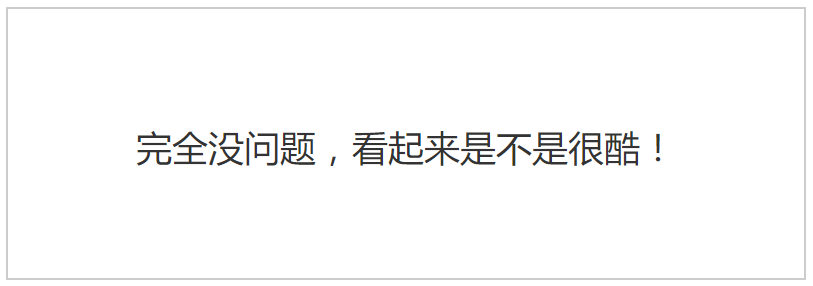this.$data = options.data; this.$el = document.querySelector(options.el);
//数据代理
Object.keys(this.$data).forEach(key => { this.proxyData(key); }); this.init(); } Mvue.prototype.init = function () { observer(this.$data);
new Compile(this);
}
Mvue.prototype.proxyData = function (key) {
Object.defineProperty(this, key, {
get: function () {
return this.$data[key] }, set: function (value) { this.$data[key] = value;
}
});
}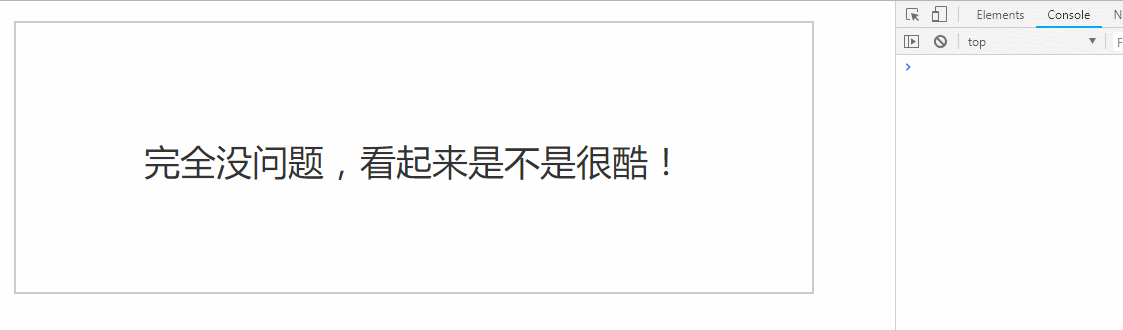#### 总结

1. 本文主要是对 Vue 双向绑定原理的学习与实现。
2. 主要是对整个思路的学习，并没有考虑到太多的实现与设计的细节，所以还存在很多问题，并不完美。
3. 源码地址，整个过程的全部代码，希望对你有所帮助。
4. 如果你觉得本文对你有帮助，欢迎转发，点赞。

posted @ 2018-10-24 17:40  六小登登  阅读(15354)  评论(4编辑  收藏  举报